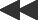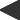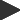basis: lyapunov-fractal derived from the bifurcation fractal, the lyapunov plots the 'lyapunov exponent' for a population model where the growth parameter varies between 2 values in a periodic manner. The Bifurcation fractal illustrates what happens in a simple population model as the growth rate increases. The Lyapunov fractal, named after Alexander Michailowitsch Ljapunow, expands that model into two dimensions by letting the growth rate vary in a periodic fashion between two values. Each pair of growth rates is run through a logistic population model and a value called the Lyapunov Exponent is calculated for each pair.The Lyapunov Exponent is calculated by adding up log | r - 2*r*x | over many cycles of the population model and dividing by the number of cycles. Negative Lyapunov exponents indicate a stable, periodic behavior. Positive Lyapunov exponents indicate chaos (or a diverging model).